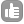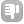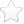1Hi, our lab uses the following relationship for the calculation of normalized CSPs from 1H-15N HSQC spectra: CSPnorm = ((0.154*Nshift)^2) + Hshift^2)^0.5 Can anyone provide the equivalent multiplier for 13C/1H normalized CSP calculations? I've been looking on the BMRB site but haven't had any success so far. Cheers. asked Oct 03 '10 at 21:231Dear Martin, these scaling factors can be calculated from the BMRB statistics (http://www.bmrb.wisc.edu/ftp/pub/bmrb/statistics/chemshifts/selected/statselprot.txt) using the method described in Mulder et al., J. Mol. Biol. (1999), 292: 111-123. In short: you calculate them from the ratio of the average variances of the nuclei. If we take the example of 1H-15N, then taking the average of the standard deviations (last column in BMRB file) for HN gives 0.646 +/- 0.055, similarly for N gives 4.132 +/- 0.709. Now giving HN a weight of 1, yields a scaling factor for nitrogen of 4.132/0.646 = 6.4 or in your formula (using it as a weight factor): 1/6.4 = 0.156. The same can be repeated for all other nuclei, for example in the case of 1H-13C for methyl groups as I have done recently [Otten et al., J. Am. Chem. Soc., (2010), 132: 2952-2960]. Calculating the same stuff for methyl groups gives: 13C = 1.567 +/- 0.22, 1H = 0.290 +/- 0.066 and a scaling factor of 5.4 (or weight of 0.185 for the carbon chemical shift). So, if you only want to look at methyl correlations you can just use this value, otherwise take one nucleus as reference and calculate the scaling factors/weights for all other nuclei. answered Oct 10 '10 at 08:50renee 561Hi Martin, There's a slight improvement on the formula you're using. Instead of the root of the sum of squares, it's better to use the root of the mean of squares. That gives a number that is directly comparable to a difference in proton frequencies. The formula you're using now is expected to be (1^2+1^2)^0.5 = sqrt(2) larger than that. Consider for example what you would do if you were following CSP from a 1H spectrum on unlabeled material, or if you would be following CSP using a HNCO, monitoring 3 frequencies. Using the root-sum-of-squares these number would have to be scaled by the inverse of the sqare of the nr of nuclei, to be comparable to a 1H-CSP, pr 1H/15N CSP. With the root-mean-square the number would remain on the same scale. 1D-1H CSP ~ dH ( = (dH^2)^0.5 ) 2D-1H/15N CSP ~ ( ( dH^2 + (cN * dN)^2 )/2 )^0.5 3D-1H/15N/13C CSP ~ ( ( dH^2 + (cN * dN)^2 + (cC * dC)^2 )/3 )^0.5 etc.. Eiso answered Oct 11 '10 at 03:33eiso 31

 toggle preview

Tags:

×4
×1

Asked: Oct 03 '10 at 21:23

Seen: 6,130 times

Last updated: Oct 11 '10 at 03:33